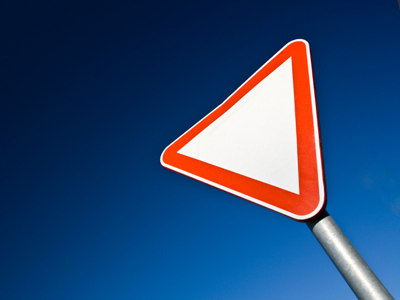The angles of a triangle total 180o.

# Position 2

This Math quiz is called 'Position 2' and it has been written by teachers to help you if you are studying the subject at middle school. Playing educational quizzes is a fabulous way to learn if you are in the 6th, 7th or 8th grade - aged 11 to 14.

It costs only \$12.50 per month to play this quiz and over 3,500 others that help you with your school work. You can subscribe on the page at Join Us

Knowing position involves being able to read the coordinates of shapes on a grid. Coordinates are used for map reading and also in knowing where to place a specific point on a grid. But how do the coordinates change when shapes are rotated or reflected? Working out the new coordinates of a rotated shape can be difficult without a pencil and paper!

See how much you remember from your math classes by trying this quiz all about position.

1.
If a triangle has a 60o angle and a 40o angle what angle is the third corner?
60o
70o
80o
90o
60o + 40o = 100o so 180o - 100o = 80o
2.
A triangle has the coordinates (1,1) (1,4) (4,1). What will the coordinates be after a quarter turn clockwise?
(1,1) (4,1) (1,-2)
(1,-1) (4,1) (1,-4)
(1,1) (4,1) (1,4)
(1,1) (4,1) (4,-4)
If you worked that out without drawing on some paper then very well done!
3.
If I turn 60o how much more do I need to turn to complete one full turn?
100o
200o
300o
400o
360o - 60o = 300o
4.
What do the angles of a triangle total?
180o
360o
200o
90o
No matter what lengths its sides are or what shape it is, the angles in a triangle always add up to 180o
5.
What is used to measure angles?
Ruler
Protractor
Tape measure
Compass
Most protractors are semi-circles divided into 180o
6.
What do we call two lines that cross one another?
Intersecting
Friends
Meeting
Crisscross
Intersecting lines meet but parallel lines never do
7.
A shape has the coordinates (5,1) (1,4) (5,6) (9,4). What shape is it?
Square
Rectangle
Rhombus
Kite
8.
A rectangle has the coordinates (2,2) (2,4) (6,4). What is the fourth coordinate?
(2,6)
(6,2)
(1,6)
(4,6)
This rectangle would have its longest sides at the top and bottom
9.
What is the intersection?
The point where two angles meet
The point where two coordinates meet
The point where two shapes meet
The point where two lines meet
Where two roads meet it is also called an intersection
10.
What is the total size of the other two angles in a right angled triangle?
30o
45o
60o
90o
180o - 90o = 90o so the other two angles must add up to that
Author:  Amanda Swift